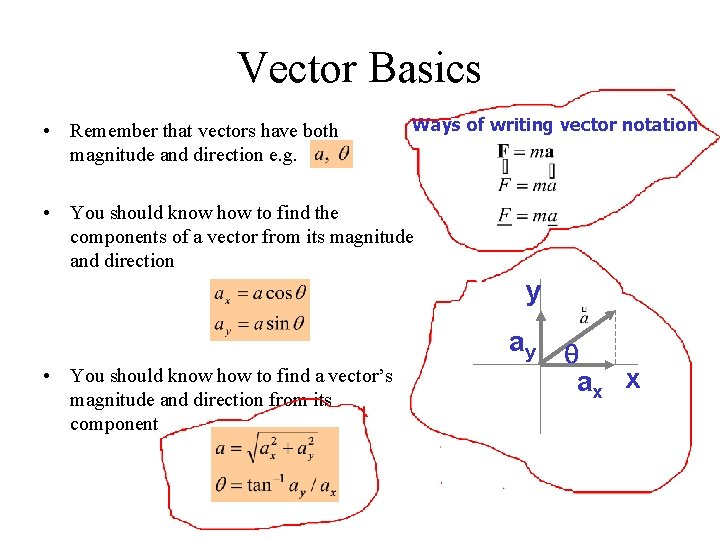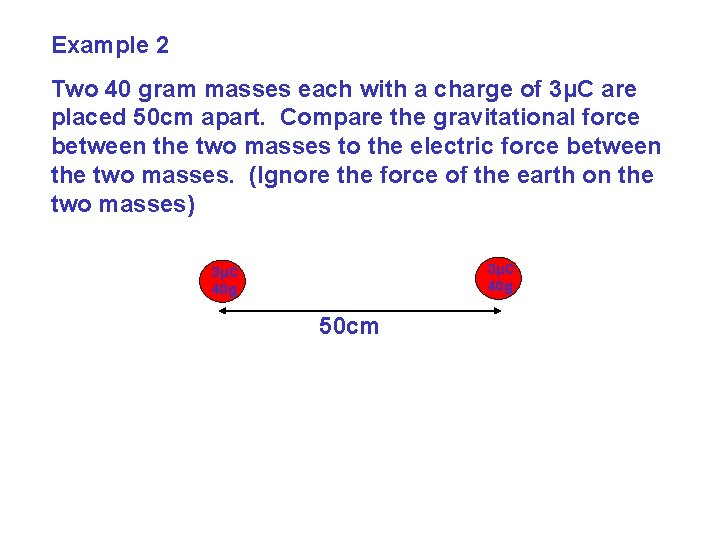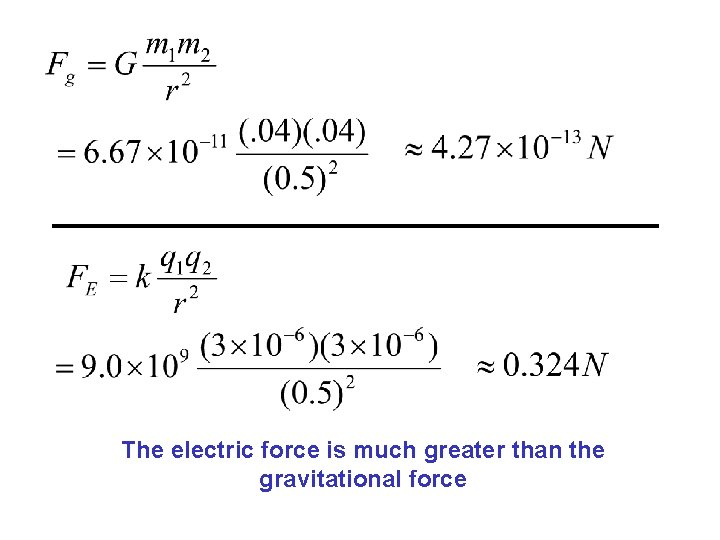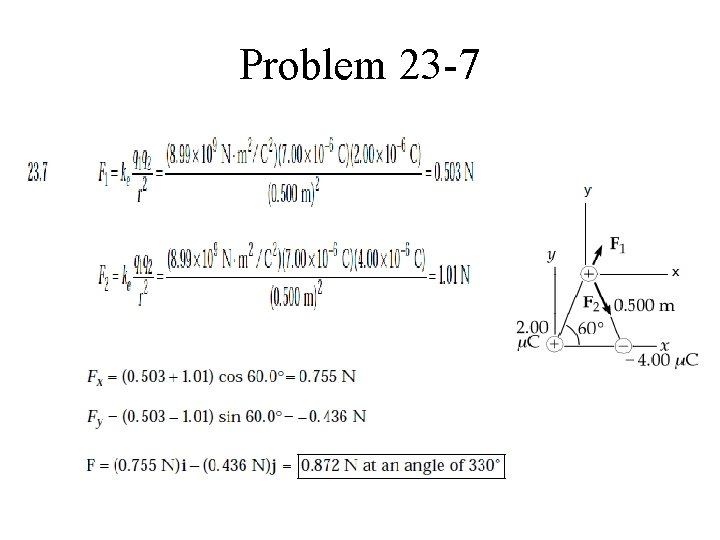# 23 3 Coulombs Law Coulombs law gives the

• Slides: 923 -3 Coulomb’s Law Coulomb’s law gives the force between two point charges: The force is along the line connecting the charges, and is attractive if the charges are opposite, and repulsive if the charges are like.23 -3 Coulomb’s Law The forces on the two charges are actionreaction forces.Vector Basics • Remember that vectors have both magnitude and direction e. g. Ways of writing vector notation • You should know how to find the components of a vector from its magnitude and direction y ay • You should know how to find a vector’s magnitude and direction from its component q ax xExample 1 Two charges are separated by a distance r and have a force F on each other. F q 2 q 1 F r If r is doubled then F is : ¼ of F If q 1 is doubled then F is : 2 F If q 1 and q 2 are doubled and r is halved then F is : 16 FExample 2 Two 40 gram masses each with a charge of 3μC are placed 50 cm apart. Compare the gravitational force between the two masses to the electric force between the two masses. (Ignore the force of the earth on the two masses) 3μC 40 g 50 cmThe electric force is much greater than the gravitational forceExample 3 Three charged objects are placed as shown. Find the net force on the object with the charge of -4μC. - 5μC 45º 20 cm 5μ C 20 cm F 145º- 4μC F 2 F 1 and F 2 must be added together as vectors.F 1 -2. 9 2. 3 cos 45≈1. 6 45º F 2 2. 3 sin 45≈1. 6 29º -1. 6 3. 31 F 1 = < - 4. 5 , 0. 0 > + F 2 = < 1. 6 , - 1. 6 > Fnet = < - 2. 9 , - 1. 6 > 3. 31 N at 209ºProblem 23 -7